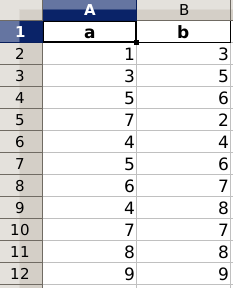# Write Excel with Pandas

Excel files can be created in Python using the module Pandas. In this article we will show how to create an excel file using Python.

Related course:
Data Analysis in Python with PandasWrite Excel
We start by importing the module pandas. From the module we import ExcelWriter and ExcelFile.
The next step is to create a data frame. In the data frame we put a list, with the name of the list as the first argument:

In this short code snippet, ‘a’ and ‘b’ are columns’. The second is the list of data the these columns will contain.

Then we write data frame to an excel file using:

The complete code:

The output of this code:Back